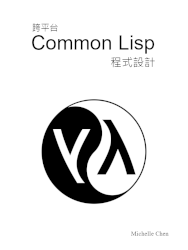#[Common Lisp] 程式設計教學：資料型態 (Data Type)

## 漸進型態 (Gradual Typing)

``````(defun fac (n)
(declare (unsigned-byte n))
(cond ((= 0 n) 1)
(t (* n (fac (- n 1))))))
``````

Common Lisp 中用來檢查型態的指令有 `declare``check-type`。兩者在行為上有一些差異，詳見下文。

## Common Lisp 的資料型態

• 布林 (boolean)
• 數字 (number)
• 字元 (character)
• 符號 (symbol)
• 列表 (list)
• 陣列 (array)
• 雜湊表 (hash table)
• 套件 (package)
• 檔案路徑 (pathname)
• 資料串流 (stream)
• 隨機狀態 (random stats)
• 函式 (function)
• 結構體 (structure)
• 類別 (class)
• Condition (註2)

(註1) 儲存 Lisp 語法的物件，用來搭配輸出入指令，如 `read`

(註2) 用於警告 (warning) 和錯誤 (error) 的物件。

## 布林 (Boolean)

Common Lisp 的布林數有兩個值，分別為 `t``nil`。前者為真 (true)，後者為偽 (false)。

## 數字 (Number)

Common Lisp 的數字資料型態如下：

• `number` (數字)
• `real` (實數)
• `rational`
• `integer` (整數)
• `fixnum`
• `bignum`
• `ratio` (有理數、分數)
• `float` (浮點數、小數)
• `short-float`
• `single-float`
• `double-float`
• `long-float`
• `complex` (複數)

### 整數 (Integer)

Common Lisp 標準規範整數可達無限大及無限小。實務上，整數的大小會受到系統資源的限制。所以，Common Lisp 可用來實作大數運算相關任務。

``````(load "common.lisp")

(defun main ()
(puts (format t "Maximum of fixnum: ~D" most-positive-fixnum))
(puts (format t "Minimum of fixnum: ~D" most-negative-fixnum))
(quit-with-status 0))

(main)
``````

## 列表 (List)

``````(assert (equal nil '()))
``````

## 陣列 (Array)

Common Lisp 的一維陣列 (one-dimensional array) 又稱為 vector。這裡的 vector 並非數學上的向量，而是指一維陣列。除了 Common Lisp 外，有些語言也用 vector 來稱呼一維陣列，像是 C++。

## 套件 (Package)

Common Lisp 的套件並不是函式庫或物件庫，而是命名空間 (namespace) 的概念。套件的目的是避開命名空間的衝突 (name collision)。

Common Lisp 預設的套件有 `:cl` (Common Lisp) 和 `:cl-user` (Common Lisp User)。在撰寫程式時，若未指定套件，會自動使用 `:cl`

## 函式 (Function)

``````(assert (notevery #'evenp '(1 2 3 4 5)))
``````

notevery 函式有兩個 (以上) 的參數，第一個參數是函式物件，第二個 (以上) 的參數是序列。本範例程式將 evenp 函式做為參數傳入。該程式的意思是列表 `'(1 2 3 4 5)` 的元素中，並非每個元素都是偶數。

## 結構體 (Structure)

Common Lisp 的結構體類似於 C 語言的結構體 (structure) 或 Pascal 的記錄 (record)，是使用者自訂型態。結構體可用來表達純量資料型態無法表達的概念，或是做為動態資料結構的基礎。

``````(defstruct point
"Point in 2-dimensional spance"
(x 0.0 :type float)
(y 0.0 :type float))
``````

## 類別 (Class)

Common Lisp 的類別是另一種使用者自訂型態。如同結構體，類別可用來表達純量資料無法表達的概念。乍看之下，類別和結構體很相似，但類別支援更多的語法特性，像是多重派發 (multiple dispatch)、多重繼承 (multiple inheritance)、MOP (Meta Object Protocol) 等。

``````(defclass point ()
((x :initarg :x
:initform 0.0
:type float)
(y :initarg :y
:initform 0.0
:type float))
(:documentation
"Point in 2-dimensional space"))
``````

## Condition

Condition 是 Common Lisp 用來處理例外 (exception) 的資料型態。例外是在程式因種種外部因素無法正確運行時，跳脫一般程式運行流程的語法機制。

## 和資料型態相關的指令

Common Lisp 支援數個和資料型態相關的指令。當程式設計者需要在程式中處理物件的資料型態時，這些指令就很方便。以下是一些常見的指令：

• typep：檢查是否符合特定型態
• check-type：檢查是否符合特定型態，不符合時引發錯誤
• subtypep：檢查是否符合特定子型態
• type-of：取得資料的型態
• deftype：定義新的資料型態

(註) predicate 是檢查物件是否為真的函式。相當於「xx 是否為真？」。

• numberp：檢查物件是否為數字
• stringp：檢查物件是否為字串
• listp：檢查物件是否為列表 (或串列)
• arrayp：檢查物件是否為陣列
• hash-table-p：檢查物件是否為雜湊表

(註)：即非負整數。

``````(defun non-negative-p (n)
(<= 0 n))

(deftype natural-number (&optional n)
`(and (satisfies integerp)
(satisfies non-negative-p)))

(defun main ()
; A floating-point number is not a natural number.
(assert (equal nil (typep 1.0 'natural-number)))
; A negative integer is not a natural number.
(assert (equal nil (typep -1 'natural-number)))
; Zero is a natural number.
(assert (typep 0 'natural-number))
; A positive number is a natural number.
(assert (typep 1 'natural-number))
(quit))

(main)
``````

``````(defun fib (n)
(declare (natural-number n))
(cond ((= 0 n) 0)
((= 1 n) 1)
(t (+ (fib (- n 1)) (fib (- n 2))))))
``````

## (選讀) `declare` 和 `check-type` 的差異

declare 指令和 check-type 指令都可用來檢查型態，但兩者在行為上有一些差異。

`declare` 實際的行為委由 Common Lisp 實作品自行決定。根據 Common Lisp 標準，Common Lisp 實作品可以忽略 `declare` 的結果。根據 optimize`safety` 設置，Common Lisp 實作品有可能把 `declare` 當成斷言 (assertion) 來用，或是僅把 `declare` 做為增進編譯器效能的標註。`declare` 指令會在編譯期運作，影響程式的優化。

``````(declaim (optimize (speed 0) (safety 3)))
``````

``````(defvar *safe-mode* nil "Validate data at runtime")

; Define `natural-number` here.

(defun fib (n)
(when *safe-mode*
(check-type n natural-number))
(cond ((= 0 n) 0)
((= 1 n) 1)
(t (+ (fib (- n 1)) (fib (- n 2))))))
``````

## 繼續深入